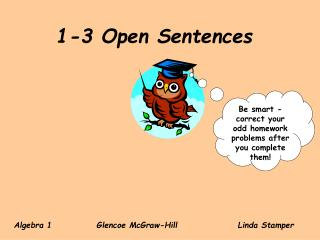DownloadDownload Presentation1-3 Open Sentences

# 1-3 Open Sentences

Télécharger la présentation## 1-3 Open Sentences

- - - - - - - - - - - - - - - - - - - - - - - - - - - E N D - - - - - - - - - - - - - - - - - - - - - - - - - - -
##### Presentation Transcript

1. 1-3 Open Sentences Be smart -correct your odd homework problems after you complete them! Algebra 1 Glencoe McGraw-Hill Linda Stamper

2. A mathematical statement with one or more variables is called an open sentence. An open sentence with an equal sign, =, is an equation. An open sentence with the symbol <, <, >, or > is an inequality.

3. An open sentence is neither true nor false until the variable/s have been replaced by specific values. The process of finding a value for the variable that results in a true sentence is called solving the open sentence. This replacement value is called a solution. The value or values of the variable that make the statement true is the solution.

4. A set of numbers from which replacements for a variable may be chosen is called a replacement set. A set is a collection of numbers. It is often shown using braces, { }. Each number in the set is called an element, or member. The solution set of an open sentence is the set of elements from the replacement set that makes the open sentence true. Replacement set {3, 4, 5, 6, 7} Solution set is {5, 6, 7}. Solution set is {5}. The value or values of the variable that make the statement true is the solution.

5. To solve an equation given a replacement set, substitute each value into the equation and simplify to determine if it makes the equation a true statement. Find the solution set for 6n + 7 = 37 if the replacement set is {4, 5, 6, 7} {5} The value or values of the variable that make the statement true is the solution.

6. Example1 Find the solution set for 5(x + 2) = 40 if the replacement set is {4, 5, 6, 7} {6} The value or values of the variable that make the statement true is the solution.

7. An inequalityis a statement formed by placing an inequality symbol between two expressions. inequality symbol meaning example <is less than4 < 8 <is less than or equal to 4 < 8 >is greater than 20 > 5 >is greater than or equal to 20 > 5 What’s the importance of is in each of the above? It represents the verb in the statement. Without it you will have an expression not a statement.

8. Beware of inequality versus subtraction. n n less than 4 − 4 expression (translation) n is less than 4 < 4 sentence n (translation) Copy in your spiral notebook! Is this an expression or a sentence? Is this an expression or a sentence?

9. To solve an inequality given a replacement set, substitute each value into the inequality and simplify to determine if it makes the inequality a true statement. Find the solution set for 30 + n > 37 if the replacement set is {5, 6, 7, 8} {7, 8} The value or values of the variable that make the statement true is the solution.

10. Example2 Find the solution set for 9 > 2y − 5 if the replacement set is {5, 6, 7, 8} {5, 6} The value or values of the variable that make the statement true is the solution.

11. Solve the equation by applying the order of operations. Write the problem. Follow rules for order of operations.

12. Solve. Example4 Example3 Example5

13. Solve. Example6

14. Solve. Example7

15. Solve. Example8

16. Solve. Example9

17. Homework 1-A5 Pages 18-20 #22-37,53-59.